Stuck on a question? Click on the solutions video below for help.

If you want to give an answer like x^2 then type x^2.

If you want to give an answer as a fraction e.g. \frac{1}{4} then type 1/4.

Any problems contact [email protected]

Practice Paper 1 Higher 2019 (Mathsgenie Version)

Q1

(3 marks)

Q2

(3 marks)

Q3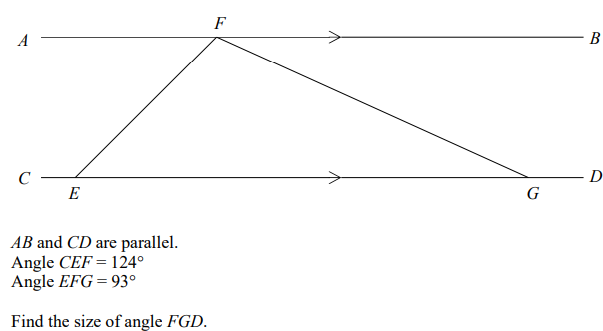(3 marks)

Q4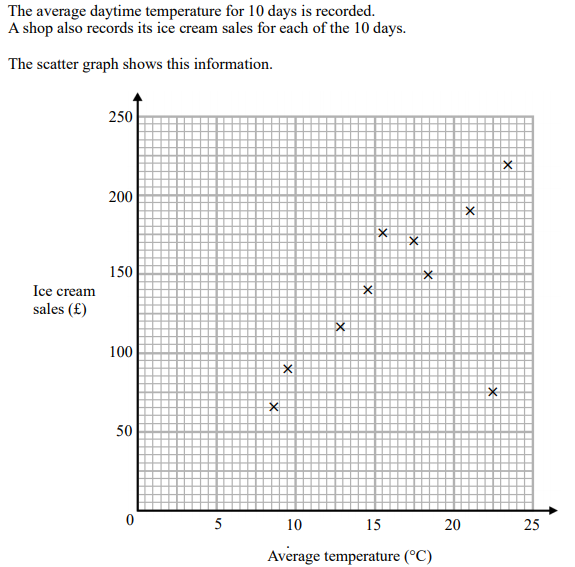a)

What type of correlation is shown?

(1 mark)

b)

Write down the coordinates of the outlier

(1 mark)

c)

On another day the temperature was 12 degrees. Estimate the ice cream sales that day.

£
(2 marks)

Q5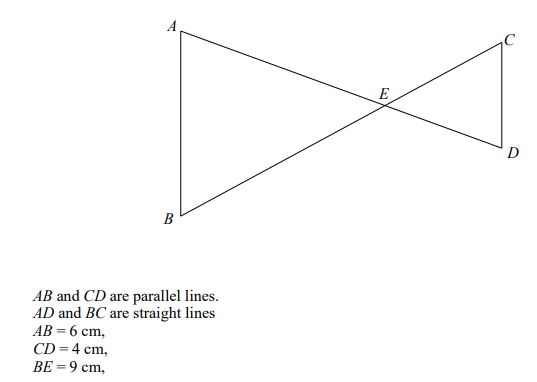Given that AE is 7.5cm, find the length of AD

(3 marks)

Q6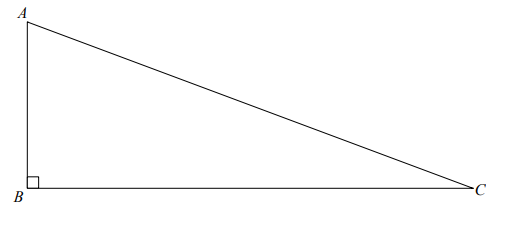AC = 13cm
BC = 12cm
AB = x
Work out x

(3 marks)

Q7

(4 marks)

Q8

x= y=
(3 marks)

Q9

A shop decreases its prices by 20%, then a further 30%. Lisa claims that this is a 50% reduction. Show tht Lisa is wrong by giving the actual reduction.

(2 marks)

Q10

In a box there are black pens, red pens and green pens.

The ratio of black pens to pens that are not black is 9:11.

The ratio of green pens to pens that are not green is 3:5.

Find the ratio of black pens to red pens to green pens.

(2 marks)

Q11

On the grid enlarge the triangle by scale factor -1.5, centre O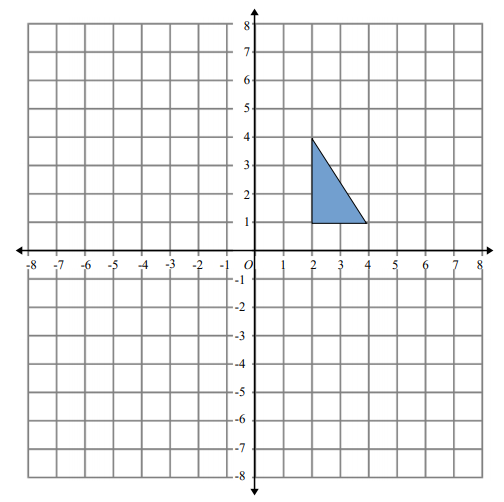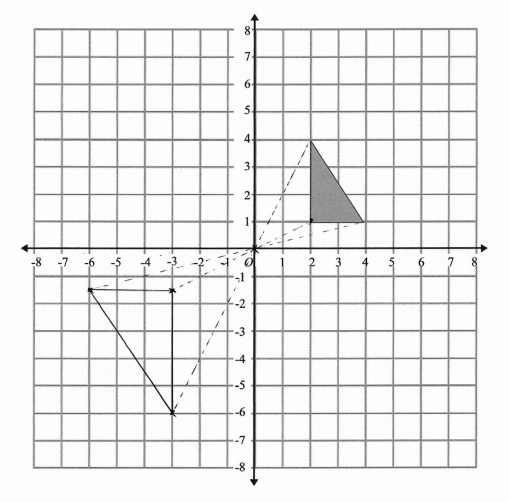(2 marks)

Q12

i)

(1 mark)

ii)

(1 mark)

iii)

(2 marks)

Q13

\lt x \lt
(3 marks)

Q14

(3 marks)

Q15

(3 marks)

Q16

Prove that the sum of any 3 consecutive even numbers is always a divisible by 6.

(3 marks)

Q17

(3 marks)

Q18

The cumulative frequency graph shows the weight, in grams, of 60 pears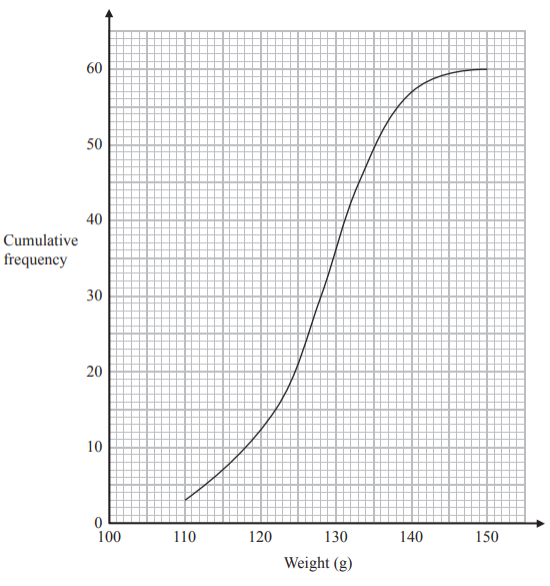The 60 pears had a minimum weight of 112 grams and a maximum weight of 149 grams. Draw a box plot to show the distribution of the weights of the pears.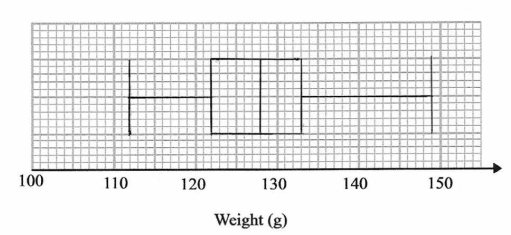(3 marks)

Q19

a is directly proportional to the square of b
When a = 12, b = 6
Find the value of b when a = 27

(3 marks)

Q20

Write \frac{sqrt{8}+sqrt{18}}{sqrt{2}-1} in the form a + b\sqrt{2}

a= b=
(3 marks)

Q21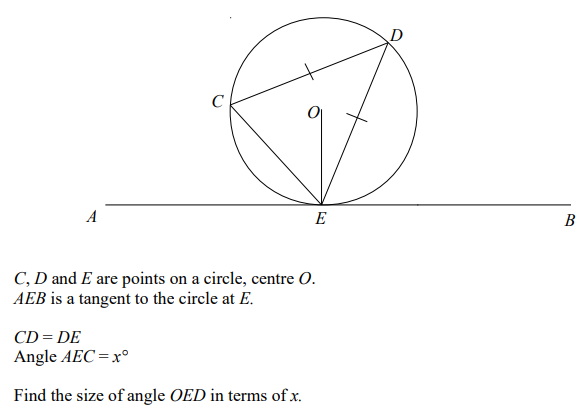(3 marks)

Q22

Line l passes through the point (2, 3).
Line 2 has the equation 4x – 2y = 3
Lines l and 2 are perpendicular.
Work out the equation of line 1.

(5 marks)

Q23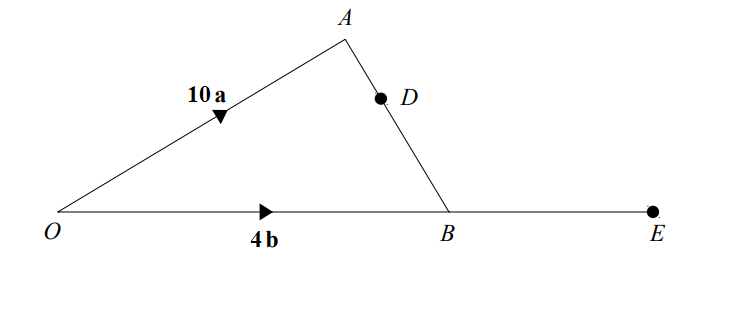Point C is the point on OA such that OC:CA = 4:1. D is the point such that AD:DB = 1:2. CDE is a straight line. Find \vec{BE}

(5 marks)

Q24

There are some red counters and some blue counters in a bag.
The ratio of red counters to blue counters is 4:1.
Two counters are removed at random.
The probability that both the counters taken are red is \frac{22}{35}
Work how many blue counters are in the bag.

(3 marks)